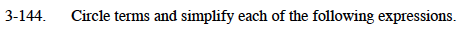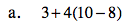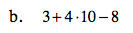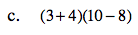### Home > MC1 > Chapter 3 > Lesson 3.4.5 > Problem3-144

3-144.
1. . Circle terms and simplify each of the following expressions. Homework Help ✎

1. 3 + 4(10 − 8)

2. 3 + 4 · 10 − 8

3. (3 + 4)(10 − 8)Refer to problem 2-132 if you need a reminder on how to simplify expressions. Remember to pay attention to parentheses.

11The method for solving this problem is the same as what you have been practicing. Before you start, how is this problem different from part (a)? This difference will greatly change the value of the expression, so be careful while simplifying.James thinks that this problem is the same as part (b), since the order of operations says that multiplication comes before addition. What do you think?